Скачать презентацию Chapter 18 Money Supply Money Demand

5d788372c1fef6a195d7aceea01edd49.ppt

• Количество слайдов: 25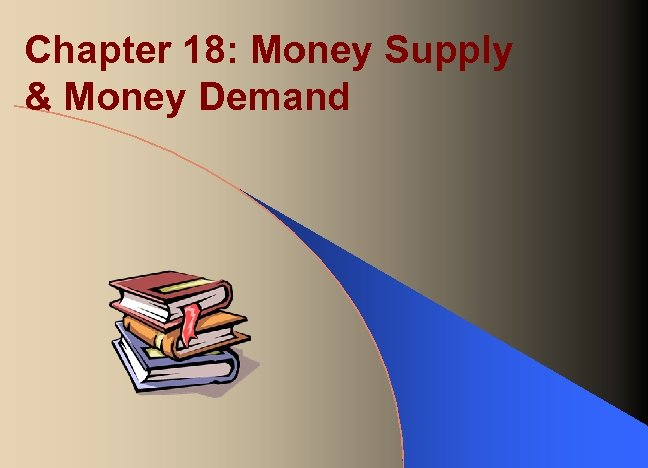Chapter 18: Money Supply & Money Demand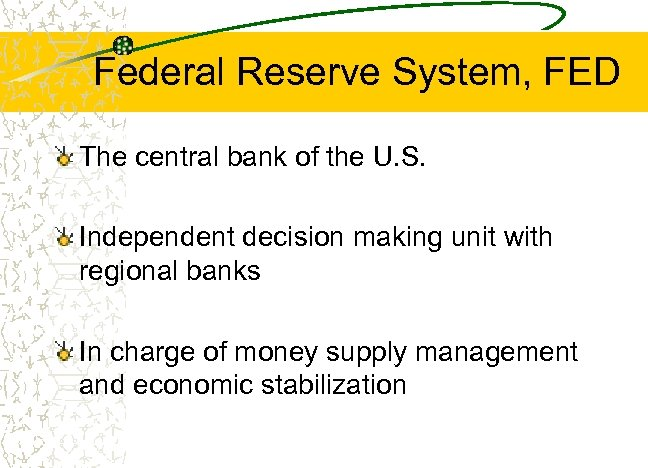Federal Reserve System, FED The central bank of the U. S. Independent decision making unit with regional banks In charge of money supply management and economic stabilization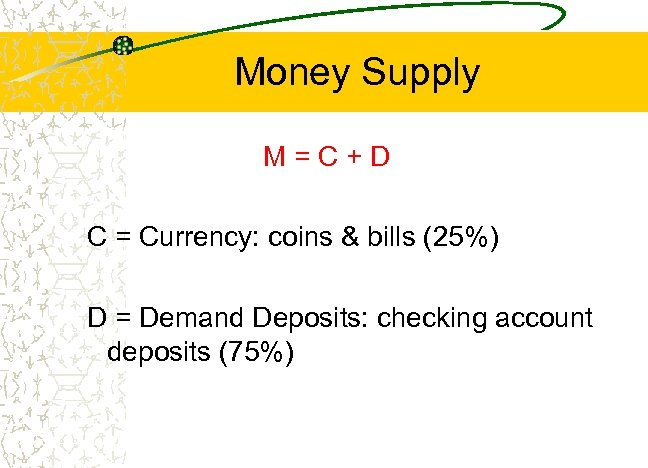Money Supply M=C+D C = Currency: coins & bills (25%) D = Demand Deposits: checking account deposits (75%)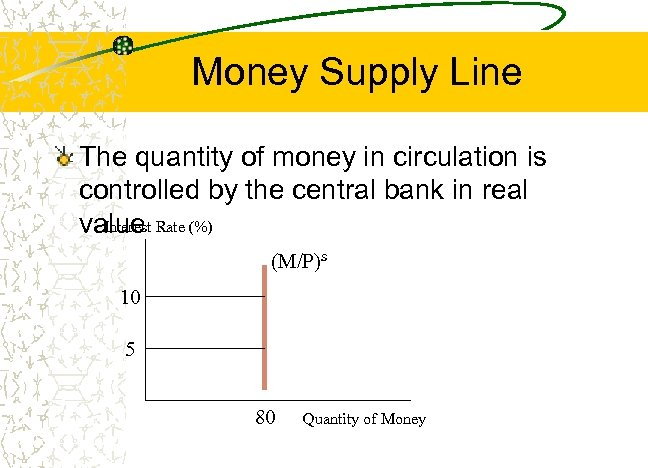Money Supply Line The quantity of money in circulation is controlled by the central bank in real Interest value Rate (%) (M/P)s 10 5 80 Quantity of Money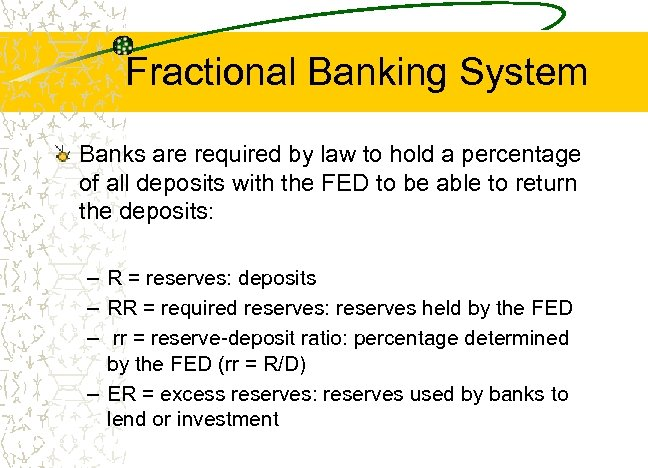Fractional Banking System Banks are required by law to hold a percentage of all deposits with the FED to be able to return the deposits: – R = reserves: deposits – RR = required reserves: reserves held by the FED – rr = reserve-deposit ratio: percentage determined by the FED (rr = R/D) – ER = excess reserves: reserves used by banks to lend or investment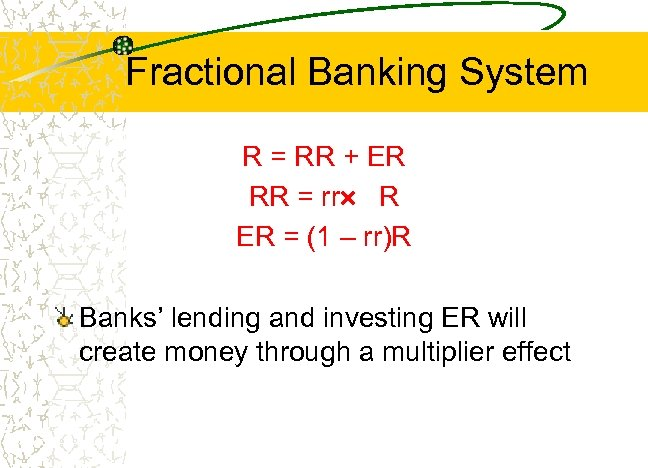Fractional Banking System R = RR + ER RR = rr R ER = (1 – rr)R Banks’ lending and investing ER will create money through a multiplier effect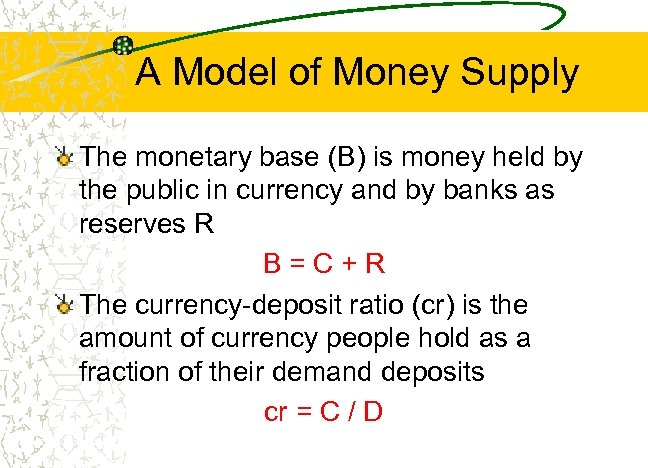A Model of Money Supply The monetary base (B) is money held by the public in currency and by banks as reserves R B=C+R The currency-deposit ratio (cr) is the amount of currency people hold as a fraction of their demand deposits cr = C / D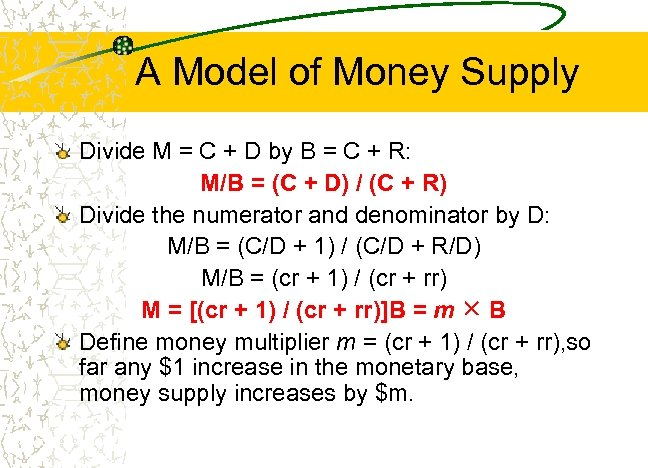A Model of Money Supply Divide M = C + D by B = C + R: M/B = (C + D) / (C + R) Divide the numerator and denominator by D: M/B = (C/D + 1) / (C/D + R/D) M/B = (cr + 1) / (cr + rr) M = [(cr + 1) / (cr + rr)]B = m B Define money multiplier m = (cr + 1) / (cr + rr), so far any \$1 increase in the monetary base, money supply increases by \$m.A Model of Money Supply Example: B = \$500 billion, cr = 0. 6 and rr = 0. 1: m=(0. 6 + 1) / (0. 6 +0. 1) = 2. 3 M = 2. 3(500) = \$1, 150 billion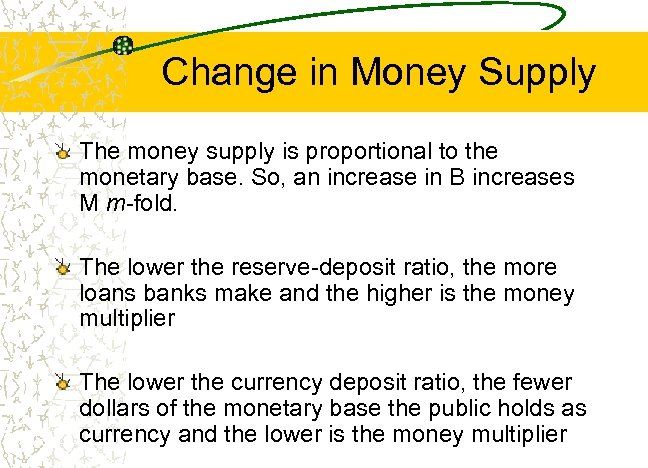Change in Money Supply The money supply is proportional to the monetary base. So, an increase in B increases M m-fold. The lower the reserve-deposit ratio, the more loans banks make and the higher is the money multiplier The lower the currency deposit ratio, the fewer dollars of the monetary base the public holds as currency and the lower is the money multiplier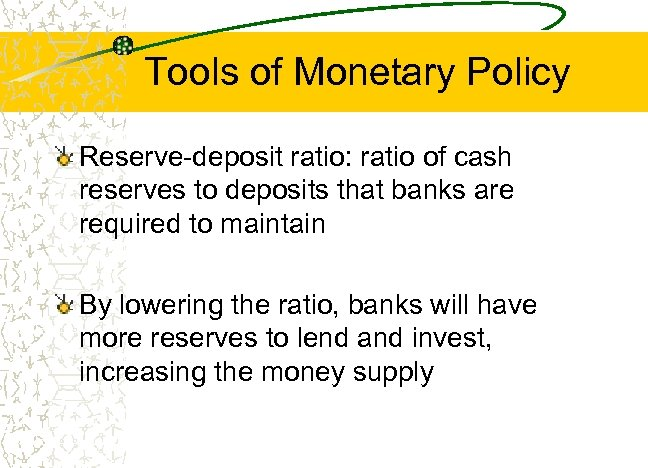Tools of Monetary Policy Reserve-deposit ratio: ratio of cash reserves to deposits that banks are required to maintain By lowering the ratio, banks will have more reserves to lend and invest, increasing the money supply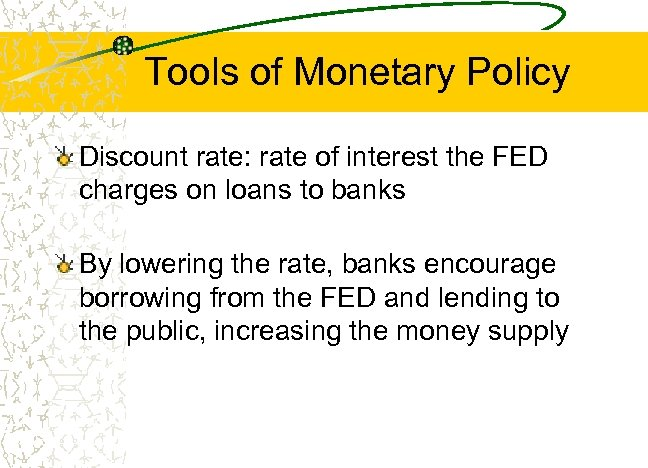Tools of Monetary Policy Discount rate: rate of interest the FED charges on loans to banks By lowering the rate, banks encourage borrowing from the FED and lending to the public, increasing the money supply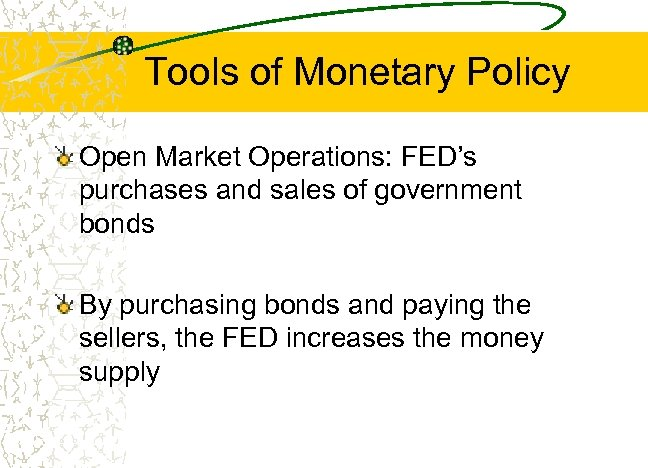Tools of Monetary Policy Open Market Operations: FED’s purchases and sales of government bonds By purchasing bonds and paying the sellers, the FED increases the money supply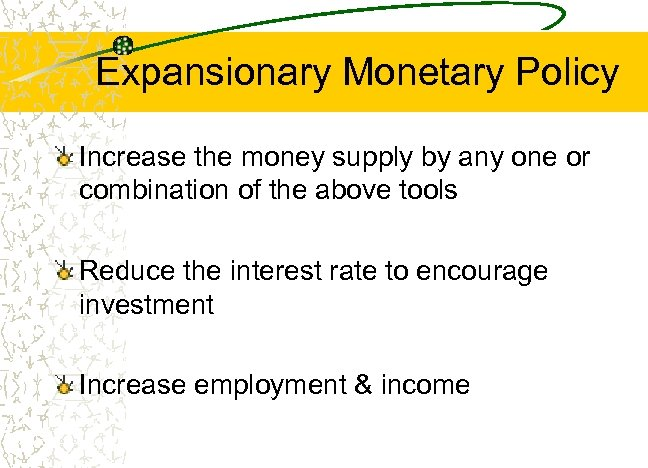Expansionary Monetary Policy Increase the money supply by any one or combination of the above tools Reduce the interest rate to encourage investment Increase employment & income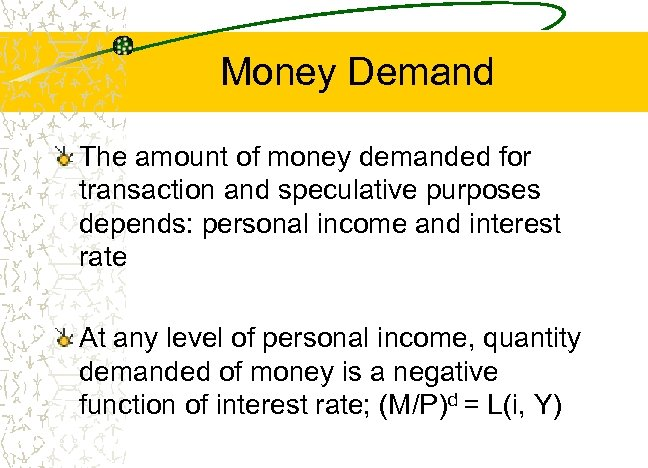Money Demand The amount of money demanded for transaction and speculative purposes depends: personal income and interest rate At any level of personal income, quantity demanded of money is a negative function of interest rate; (M/P)d = L(i, Y)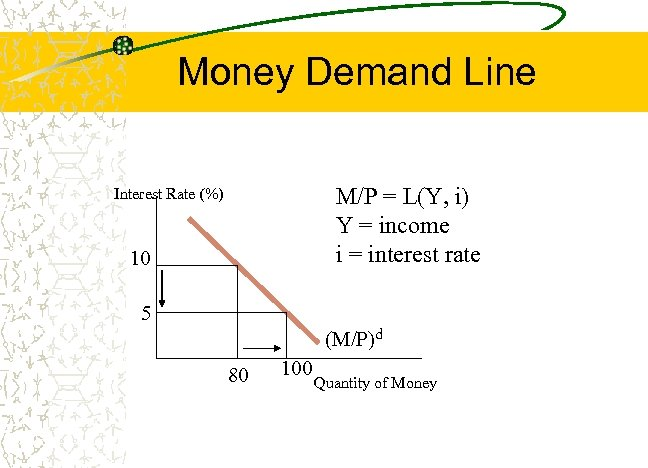Money Demand Line M/P = L(Y, i) Y = income i = interest rate Interest Rate (%) 10 5 (M/P)d 80 100 Quantity of Money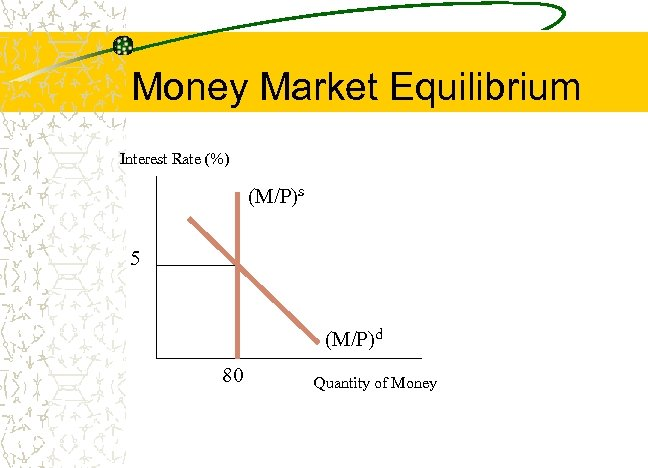Money Market Equilibrium Interest Rate (%) (M/P)s 5 (M/P)d 80 Quantity of Money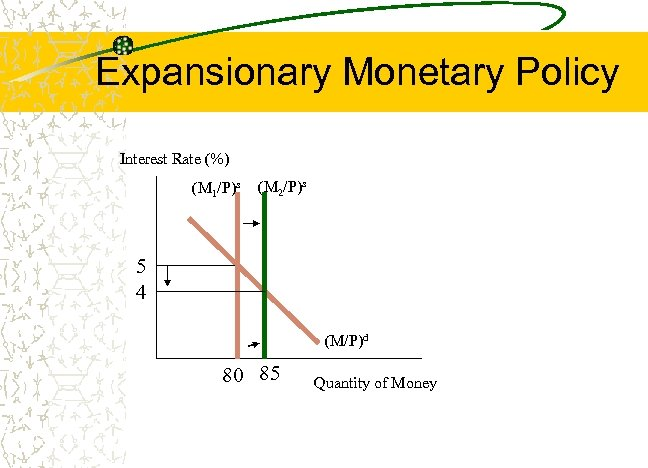Expansionary Monetary Policy Interest Rate (%) (M 1/P)s (M 2/P)s 5 4 (M/P)d 80 85 Quantity of Money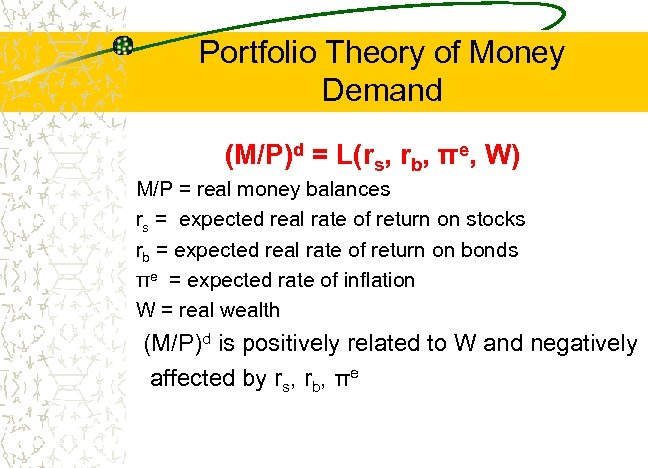Portfolio Theory of Money Demand (M/P)d = L(rs, rb, πe, W) M/P = real money balances rs = expected real rate of return on stocks rb = expected real rate of return on bonds πe = expected rate of inflation W = real wealth (M/P)d is positively related to W and negatively affected by rs, rb, πe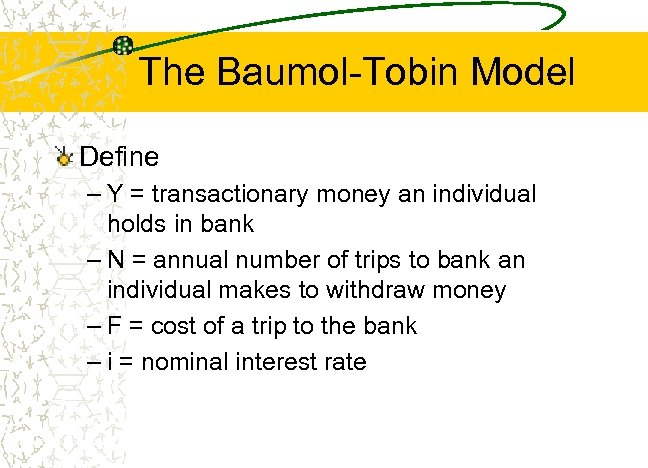The Baumol-Tobin Model Define – Y = transactionary money an individual holds in bank – N = annual number of trips to bank an individual makes to withdraw money – F = cost of a trip to the bank – i = nominal interest rate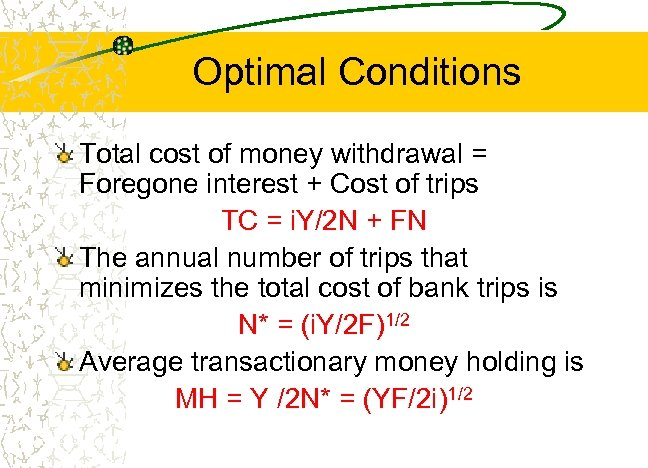Optimal Conditions Total cost of money withdrawal = Foregone interest + Cost of trips TC = i. Y/2 N + FN The annual number of trips that minimizes the total cost of bank trips is N* = (i. Y/2 F)1/2 Average transactionary money holding is MH = Y /2 N* = (YF/2 i)1/2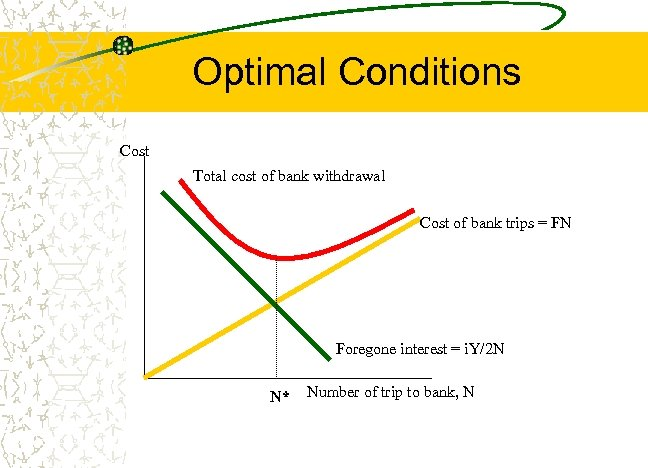Optimal Conditions Cost Total cost of bank withdrawal Cost of bank trips = FN Foregone interest = i. Y/2 N N* Number of trip to bank, N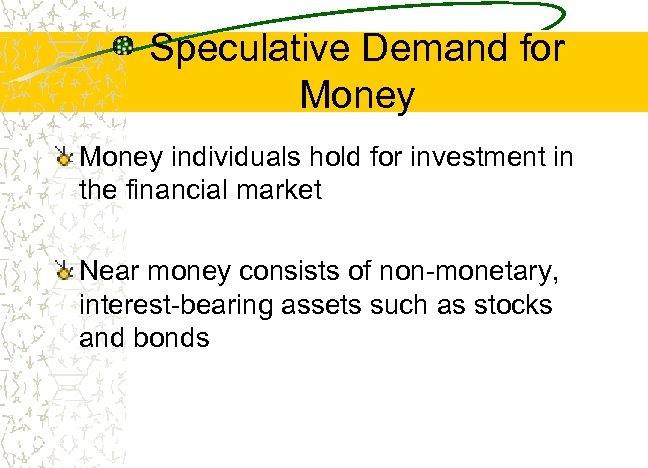Speculative Demand for Money individuals hold for investment in the financial market Near money consists of non-monetary, interest-bearing assets such as stocks and bonds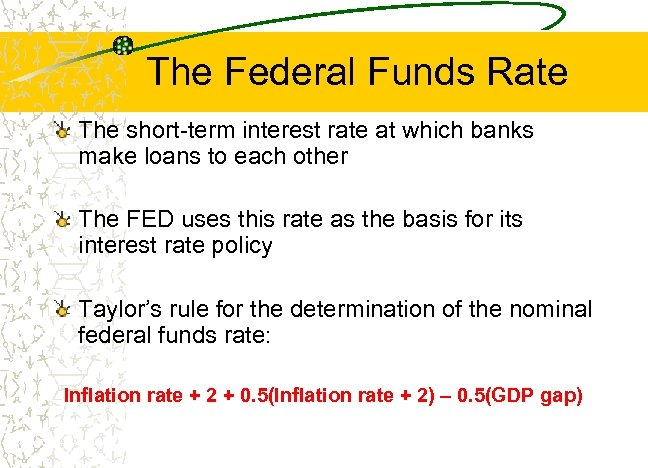The Federal Funds Rate The short-term interest rate at which banks make loans to each other The FED uses this rate as the basis for its interest rate policy Taylor’s rule for the determination of the nominal federal funds rate: Inflation rate + 2 + 0. 5(Inflation rate + 2) – 0. 5(GDP gap)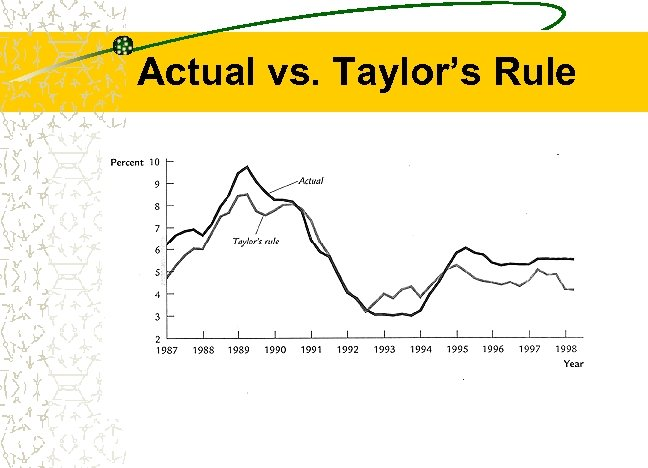Actual vs. Taylor’s Rule